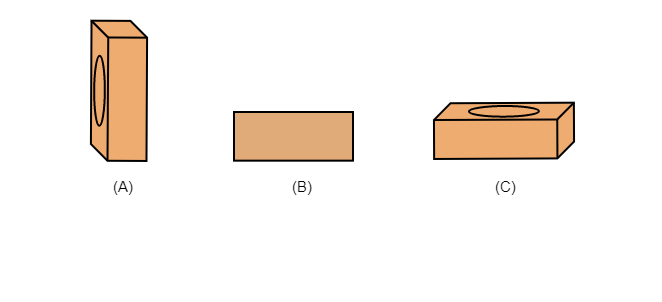# A brick is kept in three different ways on a table as shown in the figure. The pressure exerted by the brick on the table will be$(a)$. maximum in position A $(b)$. maximum in position C$(c)$. maximum in position B $(d)$. equal in all cases"

The pressure is inversely proportional to the area on which the force is being exerted. When a brick is kept on a table in $(A)$ position, the area of contact will be minimum and the pressure exerted on the table will be maximum.

So, option $(a)$ is correct.